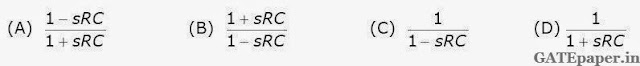### GATE 2007 ECE Video Solutions on Analog Circuits (Analog Electronics)

1. In a Transconductance amplifier, it is desirable to have
a. A large input resistance and a large output resistance
b. A large input resistance and a small output resistance
c. A small input resistance and a large output resistance
d. A small input resistance and a small output resistance

2. For the OP-AMP circuit shown in the figure, Vo isa. – 2 volts
b. – 1 volts
c. – 0.5 volts
d. + 0.5 volts

3. In the OP-AMP circuit shown, assume that the diode current follows the equation I = ISexp(V/VT). For Vi = 2 Volts, Vo = V01, and for Vi = 4 Volts, Vo = V02. The relationship between V01 and V02 isa. V02 = √2 V01
b. V02 = e2 V01
c. V02 = V01 ln2
d. V02 - V01 = VT ln2

Consider the OP-AMP circuit shown in the figure.4. The Transfer function Vo(s)/ Vi(s) is5. If Vi = V1 sin(ωt) and Vo = V2 sin(ωt+ø), then the minimum and maximum values of ø (in radians) are respectively
a. – π/2 and π/2
b. 0 and π/2
c. – π and 0
d. – π/2 and 0